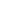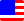Documents  >  Tutorial  >  group6
Operators of the 6th group

Purpose of this chapter.

We will learn about the equality comparison operator.

Operators of the 6th group.

Operators of the 6th group are following.

 == x == y value equality operator != x != y value inequality operator \$\$ x \$\$ y reference equality operator !\$ x !\$ y reference inequality operator

These operators are called equality comparison operators. Equality comparison operators compare the equivalent relationship of operands and return the result as a boolean value.

There are two criteria whether operands are equivalent. They are value and reference.

value equality operator

The value equality operator returns true if the left and the right operands are equal in value. Otherwise, it returns false.

For example,

bool result0 = 10 == 10; //The result is true;

bool result1 = 10 == 11; //The result is false;

bool result2 = "string" == "string"; //The result is true;

bool result3 = "string" == "strin"; //The result is false;

In the Rice, the int, long, real, string, and bool class are defined as classes that represent value. That is, the operands of the value equality operator must be one of these five classes. If you apply a value equality operator to other classes, an exception will be thrown.

Also, the classes of the left and right operands of a value equality operator must be the same. If the classes of the left and right operands are different, an exception will be thrown.(Changed in Ver 1.0.1.2)

When operands of a value equality operator are string or bool class, the classes of the left and right operands must be the same. If the classes of the left and right operands are different, an exception will be thrown.

When operands of a value equality operator are int, long or real class, You can mix these in the left and right operands.

In the above example, the value equality operator is used on the right side of the assignment statement, but in reality it will be often used as a conditional expression for if and while statements.

For example, if the variable i of the int class is defined, it is as follows.

if( i == 10 )

...

elseif( i == 11 )

...

endif

value inequality operator

The value inequality operator returns true if the left and the right operands are different in value. Otherwise, it returns false.

For example,

bool result0 = 10 != 10; //The result is false;

bool result1 = 10 != 11; //The result is true;

bool result2 = "string" != "string"; //The result is false;

bool result3 = "string" != "strin"; //The result is true;

In the Rice, the int, long, real, string, and bool class are defined as classes that represent value. That is, the operands of the value inequality operator must be one of these five classes. If you apply a value inequality operator to other classes, an exception will be thrown.

Also, the classes of the left and right operands of a value inequality operator must be the same. If the classes of the left and right operands are different, an exception will be thrown.(Changed in Ver 1.0.1.2)

When operands of a value inequality operator are string or bool class, the classes of the left and right operands must be the same. If the classes of the left and right operands are different, an exception will be thrown.

When operands of a value inequality operator are int, long or real class, You can mix these in the left and right operands.

In the above example, the value inequality operator is used on the right side of the assignment statement, but in reality it will be often used as a conditional expression for if and while statements.

For example, if the variable i of the int class is defined, it is as follows.

if( i != 10 )

...

elseif( i != 11 )

...

endif

reference equality operator

The reference equality operator returns true if the left and the right operands are same in reference. Otherwise, it returns false.

For example,

int i = 10;

int j = i;

bool result0 = i \$\$ j; //The result is true;

bool result1 = i \$\$ 10; //The result is false;

There is no limit to the operands of the reference equality operator.

In the above example, the reference equality operator is used on the right side of the assignment statement, but in reality it will be often used as a conditional expression for if and while statements.

For example, if the variable i and j of any class are defined, it is as follows.

if( i == j )

...

endif

reference inequality operator

The reference inequality operator returns true if the left and the right operands are different in reference. Otherwise, it returns false.

For example,

int i = 10;

int j = i;

bool result0 = i !\$ j; //The result is false;

bool result1 = i !\$ 10; //The result is true;

There is no limit to the operands of the reference inequality operator.

In the above example, the reference inequality operator is used on the right side of the assignment statement, but in reality it will be often used as a conditional expression for if and while statements.

For example, if the variable i and j of any class are defined, it is as follows.

if( i !\$ j )

...

endif